Courses

# Test: Ellipse- 3

## 30 Questions MCQ Test Mathematics For JEE | Test: Ellipse- 3

Description
This mock test of Test: Ellipse- 3 for JEE helps you for every JEE entrance exam. This contains 30 Multiple Choice Questions for JEE Test: Ellipse- 3 (mcq) to study with solutions a complete question bank. The solved questions answers in this Test: Ellipse- 3 quiz give you a good mix of easy questions and tough questions. JEE students definitely take this Test: Ellipse- 3 exercise for a better result in the exam. You can find other Test: Ellipse- 3 extra questions, long questions & short questions for JEE on EduRev as well by searching above.
QUESTION: 1

### An ellipse having foci at (3,3) and (-4,4) and passing though the origin has eccentricity equal to

Solution:

Ellipse passing through O (0, 0) and having foci P (3, 3) and Q (-4, 4) ,
Then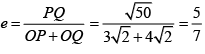QUESTION: 2

### The length of the major axis of the ellipse (5x - 10)2 + (5y  + 15)2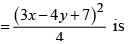Solution: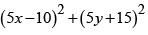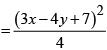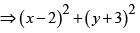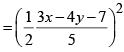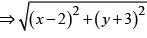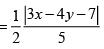is an ellipse, whose focus is (2, -3) , directrix 3x - 4y + 7 = 0 and eccentricity is 1/2.
Length of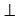from focus to directrix is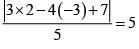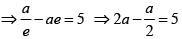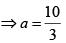So length of major axis is 20/3

QUESTION: 3

### The eccentric angle of a point on the ellipse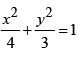at a distance of 5/4 units from the focus on the  positive x-axis, is

Solution:

Any point on the ellipse is (2 cosθ, √3 sinθ). The focus on the positive x-axis is (1,0).

Given that (2cosθ-1)2 + 3sin2 θ = 25/16
⇒ cos θ = 3/4

QUESTION: 4

From any point P lying in first quadrant on the ellipse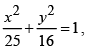PN is drawn perpendicular to the major axis and produced at Q so that NQ equals to PS, where S is a focus. Then the locus of Q is

Solution: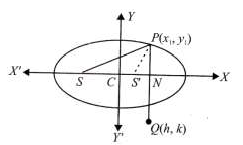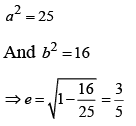Let point Q be (h,k) , where k  < 0
Given that k = SP = a + ex1 , where P (x1,y1) lies on the ellipse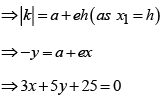QUESTION: 5

If a tangent of slope 2 of the ellipse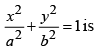is normal to the circle x2 + y2 + 4x + 1 = 0 , then the maximum value of ab is

Solution:

Let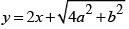be the tangent
It is passing through(-2,0)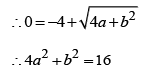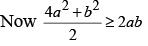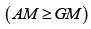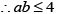QUESTION: 6

An ellipse has the points (1, -1) and (2, -1) as its foci and x +y - 5 = 0 as one of its tangents. Then the point where this line touches the ellipse is

Solution: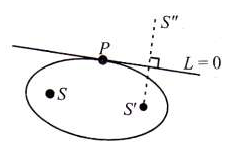Let image of S'' be with respect to x +y - 5 = 0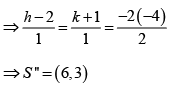Let P be the point of contact.
Because the line L = 0 is tangent to the ellipse, there exists a point P uniquely on the line such that PS + PS ' = 2a .
Since PS ' = 2a Hence, P should be the collinear with SS ''
Hence P is a point of intersection of SS '' (4x - 5 y = 9) , and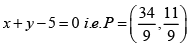QUESTION: 7

From point P (8, 27) , tangent PQ and PR are drawn to the ellipse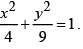Then the angle subtended by QR at origin is

Solution:

Equation of QR is T = 0 (chord of contact)
⇒ 2x + 3y = 1   .......(i)
Now, equation of the pair of lines passing through origin and points Q, R is given by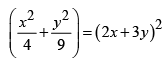(making equation of ellipse homogeneous using Eq (i)
∴ 135x2 + 432xy + 320 y2 = 0

QUESTION: 8

An ellipse is sliding along the co-ordinate axes. If the foci of the ellipse are (1,1) and (3,3), then area of the director circle of the ellipse (in sq. units) is

Solution:

Since x-axis and y-axis are perpendicular tangents to the ellipse, (0,0) lies on the director circle and midpoint of foci (2,2) is centre of the circle.
⇒ the area is 8π units.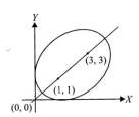QUESTION: 9

The equation of the ellipse whose axes are coincident with the co-ordinates axes and which touches the straight lines 3x - 2y - 20 = 0 and x + 6y - 20 = 0 is

Solution:

Let the equation of the ellipse be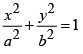We know that the general equation of the tangent to the ellipse is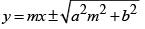......(i)
Since 2x - 2y - 20 = 0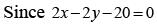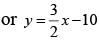is tangent to the ellipse .
Comparing with eq (i)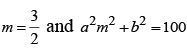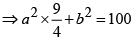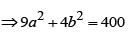......(ii)
Similarly, sinceis tangent to the ellipse, therefore  comparing with eq. …(i)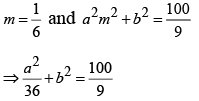⇒ a2 + 36b2 = 400.....(iii)
Solving eqs. (ii) and (iii) , we get a2 = 40 and b2 = 10 , Therefore , the required equation of the ellipse is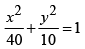QUESTION: 10

Number of point on the ellipse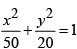from which pair of perpendicular tangents are drawn to the ellipse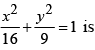Solution:

For the ellipse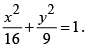Equation of director circle is x2 +y2 = 25. The director circle will cut the ellipse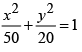at 4 points

QUESTION: 11

If maximum distance of any point on the ellipse x2 + 2y2 + 2xy = 1 from its centre be r, then r is equal to

Solution:

Here centre of the ellipse is (0,0) Let P (r cos θ, r sinθ) be any point on the given ellipse then r2 cos2θ + 2r2 sin2θ + 2r2 sin θ cos θ = 1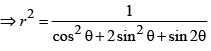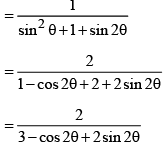QUESTION: 12

The equation of the line passing through the centre and bisecting the chord 7x +y -1 = 0 of the ellipse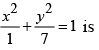Solution:

Let (h,k) be the midpoint of the chord 7 x +y -1 = 0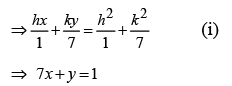Represents same straight line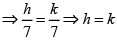⇒ Equation of the line joining (0,0) and (h,k)  is y - x = 0.

QUESTION: 13

Eccentricity of ellipse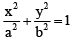such that the line joining the foci subtends a right angle only at two points on ellipse, is

Solution: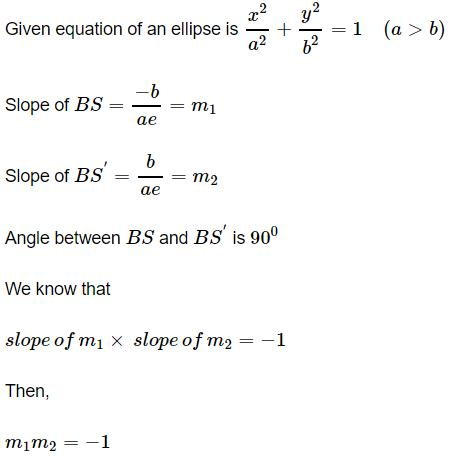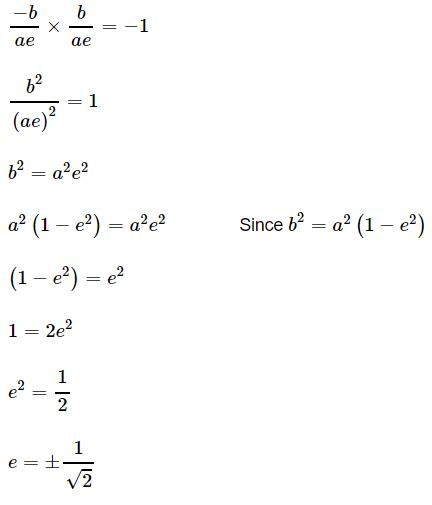QUESTION: 14

The tangent at the point ‘a’ on the ellipsemeets the auxiliary circle in two points which subtends a right angle at the centre, then the eccentricity ‘e’ of the ellipse is given by the equation :

Solution: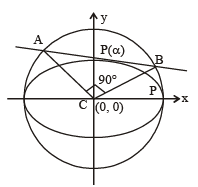Equation of auxillary circle is x2 + y2 = a2
Let P is (acosa,bsina)

Equatio of AB is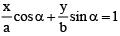bcosα.x+a sinαy = ab
To get combined equation of CA and CB, homogenize equation of circle with equation (i),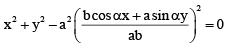b2x2 + b2y2 - (bcosα.x+a sinα.y)2 = 0
since ∠BCA = 90°
∴ coefficient of x2 + coefficient of y2 = 0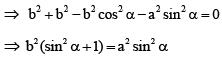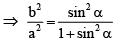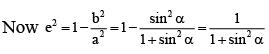QUESTION: 15

The number of values of c such that the straight line y = 4x+ c touches the curve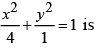Solution:

For given slope there exists two parallel tangents to ellipse. Hence, there are two values of c .

QUESTION: 16

If tangents are drawn to the ellipse x2 + 2y2 = 2, then the locus of the midpoint of the intercept made by the tangent between the coordinate axes is

Solution:For any tangent to ellipse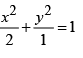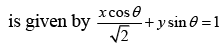Using midpoint formula, we have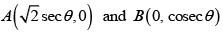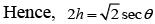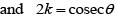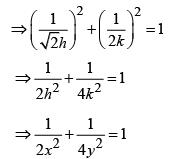QUESTION: 17

An ellipse with major and minor axes, 6√3 and 6 respectively slides along the coordinate axes and always remains confined in the first quadrant. If the length of arc described by the centre of the ellipse is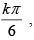then the value of k is

Solution:
QUESTION: 18

Consider the ellipse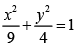and the parabola y2 = 2x. They intersect at P and Q in the first and fourth quadrants respectively. Tangents to the ellipse at P and Q intersect the x-axis at R and tangents to the parabola at P and Q intersect the x-axis at S. If the area of quadrilateral PQRS, is λ then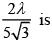Solution:

Area of quadrilateral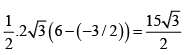QUESTION: 19

The area of the quadrilateral formed by the tangents at the end points of latus rectum of the ellipse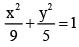is k then k/9 is

Solution:
QUESTION: 20

The length of the focal chord of the ellipse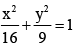which is inclined to x – axis at an angle 45° is λ, then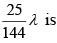Solution:
QUESTION: 21

Q is a point on the auxiliary circle corresponding to the point P of the ellipse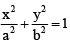If T is the foot of the perpendicular dropped from the focus S on to the tangent to the auxiliary circle at Q then the ΔSPT is :

Solution:

Tangent at Q is x cos θ + y sin θ = a
ST = |a e cosθ - a| = a(1 - e cos θ)
Also SP = e PM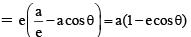ST = SP ⇒ isosceles.

QUESTION: 22

Let x and y satisfy the relation x2 + 9y2 - 4x + 6y + 4 = 0, then maximum value of the expression (4x - 9y),

Solution:

Given equation is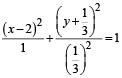Let x - 2 = cos θ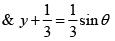Then 4x - 9y = 11+ 4 cosθ - 3sinθ

QUESTION: 23

A rectangle ABCD has area 200 square units. An ellipse with area 200π passes through A and C and has foci at B and D, then

Solution:
QUESTION: 24

A tangent to the ellipse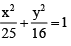at any point P meet the line x = 0 at a point Q. Let R be the image of Q in the line y = x, then circle whose extremities of a diameter are Q and R passes through a fixed point. The fixed point is

Solution:

Equation of the tangent to the ellipse at P (5 cos θ , 4 sin θ) is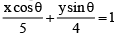It meets the line x = 0 at Q (0, 4 cosec θ)
Image of Q in the line y = x is R (4 cosec θ , 0)
∴ Equation of the circle is
X (x – 4 cosec θ) + y(y – 4 cosec θ) = 0
i.e. x2 + y2 – 4 (x + y) cosec θ = 0
∴ each member of the family passes through the intersection of x2 + y2 = 0 and x + y = 0
i.e. the point (0, 0).

QUESTION: 25

If the curve x2 + 3y2 = 9 subtends an obtuse angle at the point (2α, α), then a possible value of α2 is

Solution:

The given curve is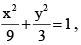whose director circle is x2 + y2 = 12. For the required condition (2α, α) should lie inside the circle and outside the ellipse i.e.,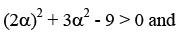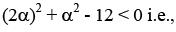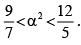QUESTION: 26

Let S = 0 be the equation of reflection of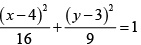about the line x – y – 2 = 0. Then the locus of point of intersection of perpendicular tangents of S is

Solution:

(x - 5)2 + (y - 2)2 = 16 + 9

QUESTION: 27

Any ordinate MP of a ellipse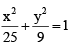meets the auxiliary circle in Q, then locus of point of intersection of normals at P and Q to the respective curves, is

Solution:

Equation of normal to the ellipse at ‘P’ is
5x sec θ – 3y cosec θ = 16 …(1)
Equation of normal to the circle x2 + y2 = 25 at point Q is –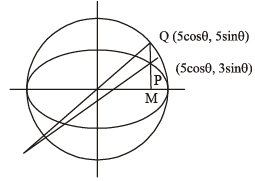y = x tan θ
Eliminating θ from (1) & (2). We get x2 + y2 = 64.

QUESTION: 28

The radius of the largest circle whose centre at (-3,0) and is inscribed in the ellipse 16x2 + 25y2 = 400 is

Solution:
QUESTION: 29

The line passing through the extremely A of the major axis and extremity B of the minor axis of the ellipse x2 + 9y2 = 9 meets its auxiliary circle at the point M . Then the area of the triangle with vertices at A,M and the origin O is

Solution:

Equation of line AM is
x +3y -3 = 0
Perpendicular distance of line from the origin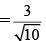Length of AM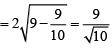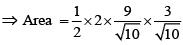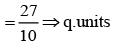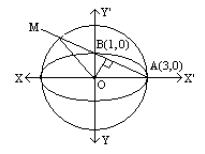QUESTION: 30

Coordinate of the vertices B and C of triangle ABC are respectively (2, 0) and (8, 0). The vertex ‘A’ is varying in such a way that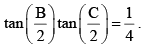Then the locus of ‘A’ is an ellipse whose major axis is of length.

Solution:

Let BC = a, CA = b & AB = c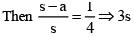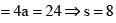∴ b + c = 2s – a = 10.
Locus of A is an ellipse with major axis of length 10